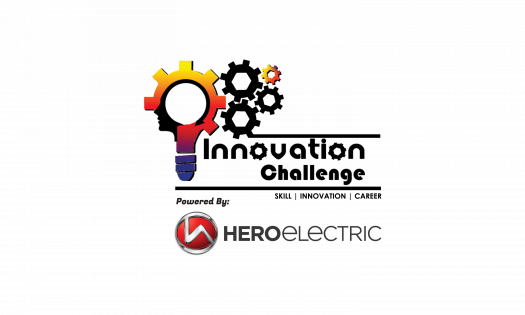# Hero Electric Innovation Challenge Online Test Assessment

30 Questions | Total Attempts: 180SettingsCreate your own QuizISIE INDIA & HERO Electric are providing comprehensive and immersive platform for creating skilled engineers, in order to new & re-assigned engineers to become proficient and productive in a short period of time. The "HERO INNOVATION CHALLENGE" will develop the skills required by the OEM’s and Tier 1 companies during their graduation time and will assist them in getting career opportunity in core industries of respective field of passion. It will enhance students for getting expertise into the specific domain by having the theoretical and practical (360 Degree) exposure.

• 1.
The energy Density of Conventional Lead acid Batteries are ----------that of Modern Lithium Ion Battery.
• A.

Higher

• B.

Lower

• C.

Same

• D.

None of these

• 2.
What is the Unit  for the Capacity of Battery?
• A.

Watt

• B.

Ampere-Hour

• C.

Ohm

• D.

Ampere

• 3.
The Watt-Hour of a Battery is Calculated by_______.
• A.

V/Ah

• B.

I*Ah

• C.

V*Ah

• D.

Ah/V

• 4.
If  25Ah Battery is Discharged by a Load of 500 Watt rated at 48v, What will be the Backup in Hours
• A.

2.4 Hours

• B.

2.6 Hours

• C.

2.7 Hours

• D.

3 Hours

• 5.
If for a 20Ah battery the C-rate is 0.5C, What is recommended Discharge Current that we should  Draw from that Battery.
• A.

10 A

• B.

11 A

• C.

40 A

• D.

15 A

• 6.
The BMS of the battery is required for
• A.

Protection

• B.

Cell Balancing

• C.

Both (a) and (b)

• D.

None of the above

• 7.
Which of the following is not a Li-ion Chemistry.
• A.

NMC

• B.

LFP

• C.

VRLA

• D.

LMO

• 8.
For a Battery Pack, if 14 cells of 3.7v 24 Ah are connected in series, what will be the resultant Voltage and Ah of Battery Pack.
• A.

51v 24Ah

• B.

51v 48Ah

• C.

60v 12Ah

• D.

51v 12Ah

• 9.
Which of the following terms are related to Battery
• A.

SOC

• B.

SOH

• C.

DOD

• D.

All of the above

• 10.
If a Motor rated at 48v draws 17A current from the battery, the Mechanical  output of the motor at 85% efficiency is
• A.

816 Watt

• B.

694 Watt

• C.

690 Watt

• D.

800 Watt

• 11.
Which of the Following sensors are used in BLDC motor for detecting Direction
• A.

Hall sensor

• B.

Torque Sensor

• C.

Accelerometer

• D.

None of the above

• 12.
Which certifying Body in India certifies Electric Vehicles?
• A.

ARAI

• B.

ICAT

• C.

BIS

• D.

Both a & b

• 13.
In Electric Vehicles DC-DC converter is  used for
• A.

Lights

• B.

Controlling Motor

• C.

Both (a) and (b)

• D.

None of the above

• 14.
If the Wheel rpm of  a scooter is 470rpm, what will be the Speed assuming a tyre radius of 201mm
• A.

35.5kmph

• B.

37.5 kmph

• C.

40kmph

• D.

45kmph

• 15.
The full form of MCB is
• A.

Mono Circuit Breaker

• B.

Miniature Circuit Breaker

• C.

Multi Circuit Breaker

• D.

None of the above

• 16.
Which Scheme in India was created for promoting electric Vehicles?
• A.

SWAYAM

• B.

FAME

• C.

Both (a) and (b)

• D.

None of the above

• 17.
Two wheeler safety norms in the current year include following
• A.

ABS

• B.

CBS

• C.

Day Light running

• D.

All of above

• 18.
CVT Transmission has following benefits
• A.

Acceleration

• B.

Good cornering

• C.

Good braking

• D.

• 19.
LED Lamps used in Automotive for following
• A.

Low Power consumption

• B.

Day time Running

• C.

Style

• D.

All of above

• 20.
The Indian driving cycle for 2 & 3 Wheeler have speed capping at
• A.

90 kmph

• B.

50 kmph

• C.

42 kmph

• D.

140 kmph

• 21.
Vehicle weight has an impact on
• A.

Power consumption

• B.

Braking

• C.

Pickup

• D.

All above

• 22.
Higher Voltage in Automotive has following problems
• A.

Higher cable cross section

• B.

EMI/ EMC

• C.

High Shock value

• D.

Non of the above

• 23.
Ola / Uber operate on following
• A.

GSM & GPS

• B.

RFID

• C.

Bluetooth

• D.

E Sim

• 24.
Following cars have online Fuel Economy
• A.

Swift

• B.

Honda

• C.

I10

• D.

Figo

• 25.
Speedometer in most of 2 Wheeler has following measurement
• A.

GPS/GSM

• B.

Wheel Speed

• C.

Gear Speed

• D.

Fuel Flow

Related TopicsBack to top
×

Wait!
Here's an interesting quiz for you.